## Saturday, August 11, 2007

### Irodov Problem 1.76I will solve this problem using two methods i) using conservation of energy and ii) newton's laws just because I love it when two different elegant approaches of explaining the world yield the same results.

Solution Using Energy Conservation
------------------------------------------- Let us first understand how the two masses move in relation to each other. The different sections of the string are marked as AB, CD, EF are depicted in the figure. Suppose, that the mass M moves down by x units, the moving pulley moves down by x units too. Thus, AB increases to AB' = AB + x. Also CD' = CD + x. Now the entire length of the string must be conserved thus AB + CD + EF = AB' + CD' + EF'. Thus, EF - EF' = 2x. In other words, if M
moves up by x units, then m moves by down 2x units. Thus, at any moment m moves twice as fast upwards as M moves downwards.

Initially the potential energy of the system is Mgh since the and the kinetic energy is 0 since none of the bodies are moving. This is total energy of the system and will be conserved until all the bodies are moving freely. When the mass M reaches the ground let the velocity of the mass be v. The velocity of m at this point will be 2v moving upwards. Also by this time the mass m moves up a distance of 2h. At this time, the mass M has completely lost all its potential energy while the mass m gains a potential energy of mg(2h). Thus we have,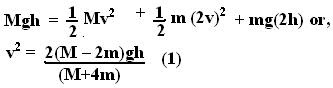The kinetic energy of m when M hits the floor is given by,As soon as M hits the floor it stops abruptly and looses all its kinetic energy immediately - this energy is completely absorbed by the floor. From this point onwards, the mass m starts to move freely under the influence of only gravity and its total energy is conserved. Let H be the height m travels up from this point onwards before it starts to fall down under the influence of gravity. At the highest point all the kinetic energy has been converted into potential energy. Thus,The total height is given by 2h + H given by,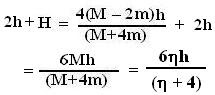Solution Using Newton's Laws
------------------------------------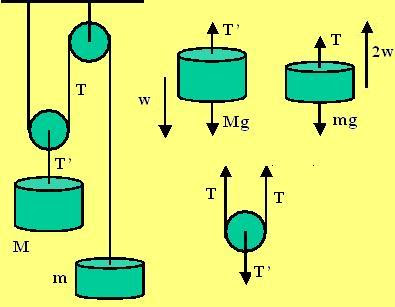Let the tension in the string holding M be T' and let the tension in the string holding m be T. Let the acceleration of M be w downwards then the acceleration of m will be 2w upwards.

Forces on M:
There are two forces acting on M, i) the tension in the string holding it T' and the ii) the force of gravity Mg. The dynamics is given by,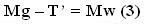Forces on m: There are two forces acting on m, i) the tension in the string T and ii) the force of gravity mg. The dynamics are thus given by,Forces acting on the moving pulley: There are three forces acting on the moving pulley, i) the tension T in the two parts of the string holding the mass m and ii) the tension T' in the string holding the mass M. The Pulley accelerates same as M at a rate w downwards and its mass is 0. Thus we have,Now we have all the information need to solve for the system. From (5) and (3) we have,From (4) and (6) we obtain,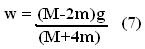The acceleration of the mass m is given by 2w. At the time mass M hits the floor, m has moved
2h units upwards. Its velocity at that time can be obtained asAfter this point there is not tension in any of the strings and the mass m moves freely under the influence of gravity thus it decelerates at a rate g. The extra height traveled by m under this influence of gravity is given by,Thus, the total height reached by m when it starts to fall down is given by,Isn't it nice that law of conservation of energy and newton's laws are in complete agreement!Anonymous said...

Sir,
CAN u plz explain why there is no tension in any of the strings as the block m1 hits the floor.....Y only it decelerates with g?? y not there is no tension in the string above it???? plz explain........

Krishna Kant Chintalapudi said...

Suppose you hang a heavy ball to a rubber band, the rubber band will be stretched. This stretching is due to the tension in the band that is supporting the ball.

As soon as you put the ball on the floor, the rubber band will not stretch (loose all its tension) any more since now the ground is supporting the ball - the rubber band is no longer needed.

Hope this helps...Anonymous said...

ok i got it thank u........Anonymous said...

i hav 2 doubts plz help me
1)u say w2=2 w1 by looking into the displacement of both masses.
does that mean aceeleration is directly proportional to the displacement?
why is that so?

2)how do we find out that M has gone and hit the floor?what if the system reached equilibrium somewhere in the middle

Krishna Kant Chintalapudi said...

1) Say that the motion of two bodies is related as follows: if m1 moves a distance of x1 then m2 must move a distance of 2x2. So

x1 = 2x2

Then differentiating their distances two times with respect to time we have,

d^2 x1/dt = 2 d^2 x2/dt or

w1 = 2w2

2)If the bodies are constantly accelerating they cannot come to an equilibrium position.Anonymous said...

it would be very helpful for students if you publish other solutions also because the solutions you give are amazingAnonymous said...

You r d best....... thanks a lotAnonymous said...

U r d best